## 哈希表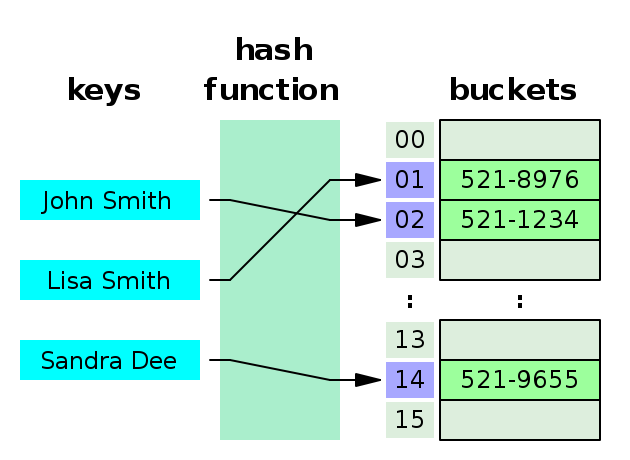• 哈希值 = 哈希函数（键）
• 索引值 = 哈希值 % 哈希表长度

## 冲突解决方法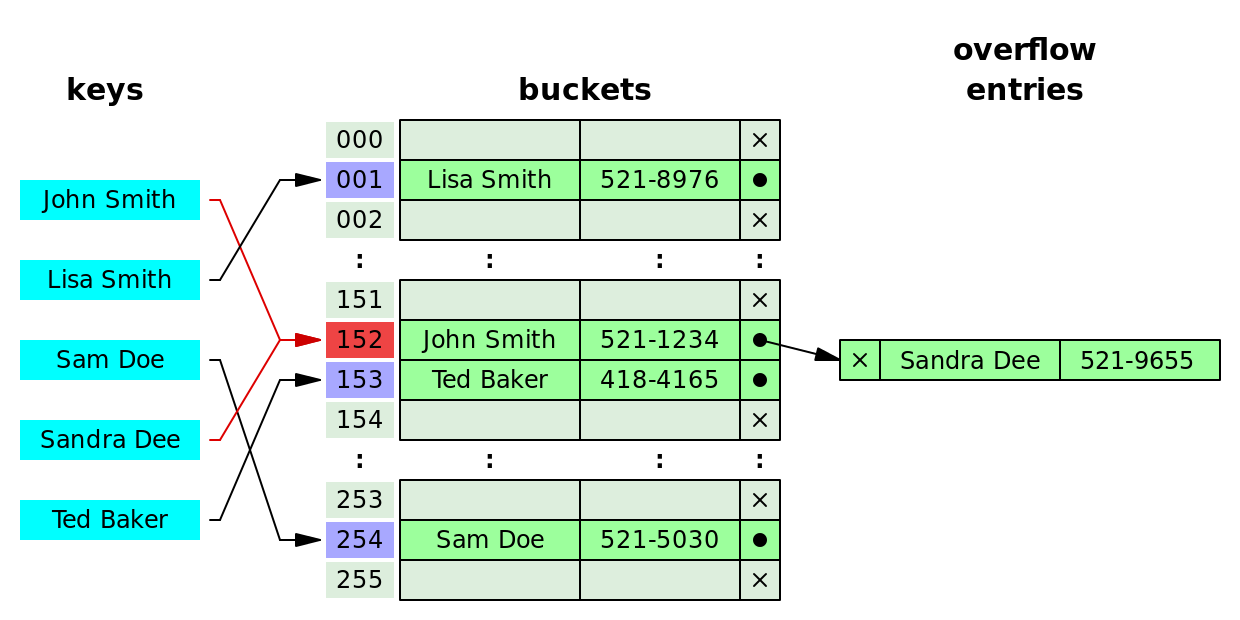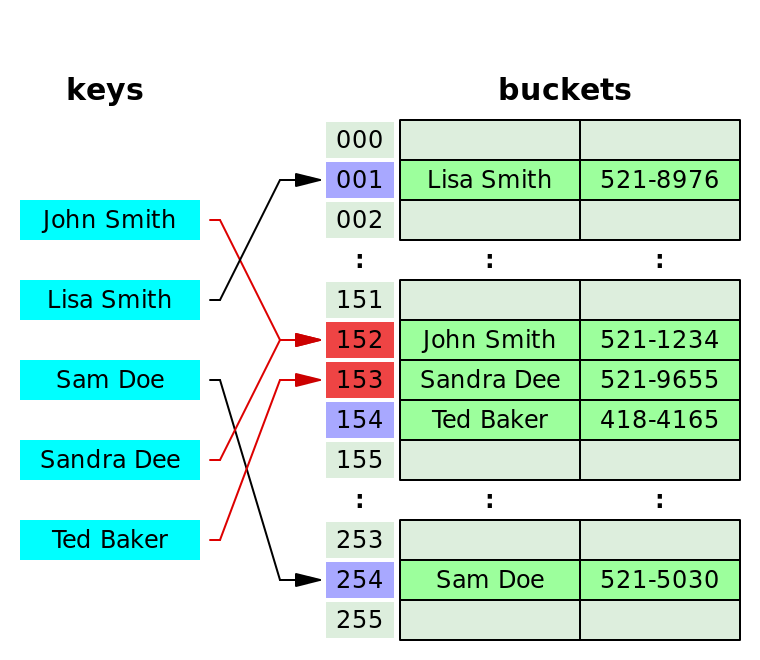## 性能• $n$ 哈希表中的条目数。
• $k$ 桶的数量。

• 缓存丢失。随着负载因子的增加缓存丢失数量上升，而搜索和插入性能会因此大幅下降。
• 扩容重新哈希。调整大小是一项极其耗时的任务。设置合适的负载因子可以控制扩容次数。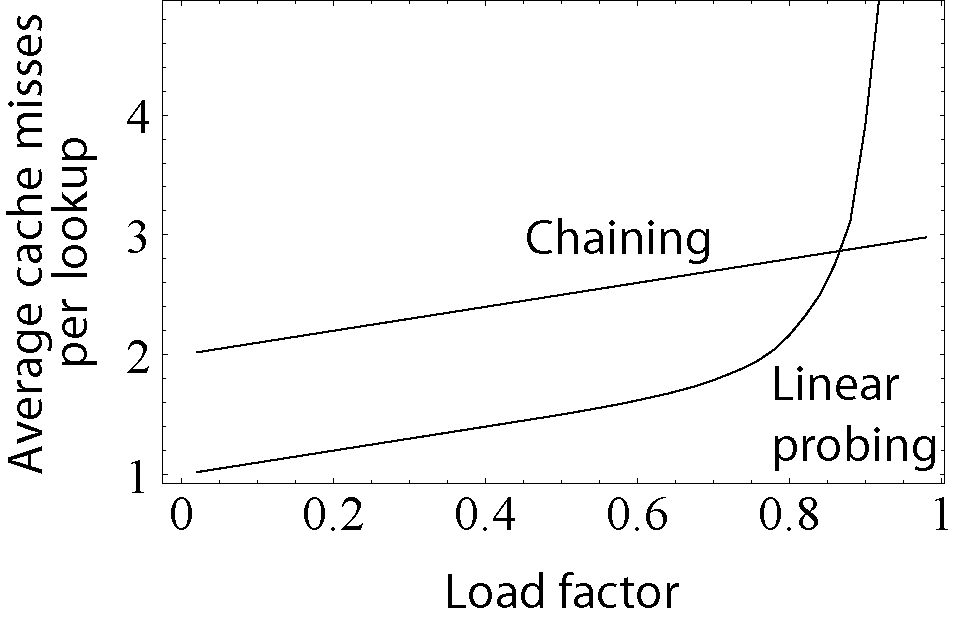## HashMap

static final int hash(Object key) {   //jdk1.8 & jdk1.7
int h;
// h = key.hashCode()  取hashCode值
// h ^ (h >>> 16)      将高16位与低16位进行异或
return (key == null) ? 0 : (h = key.hashCode()) ^ (h >>> 16);
}

// jdk1.7
static int indexFor(int h, int length) {
return h & (length-1);
}
// jdk1.8
(n - 1) & hash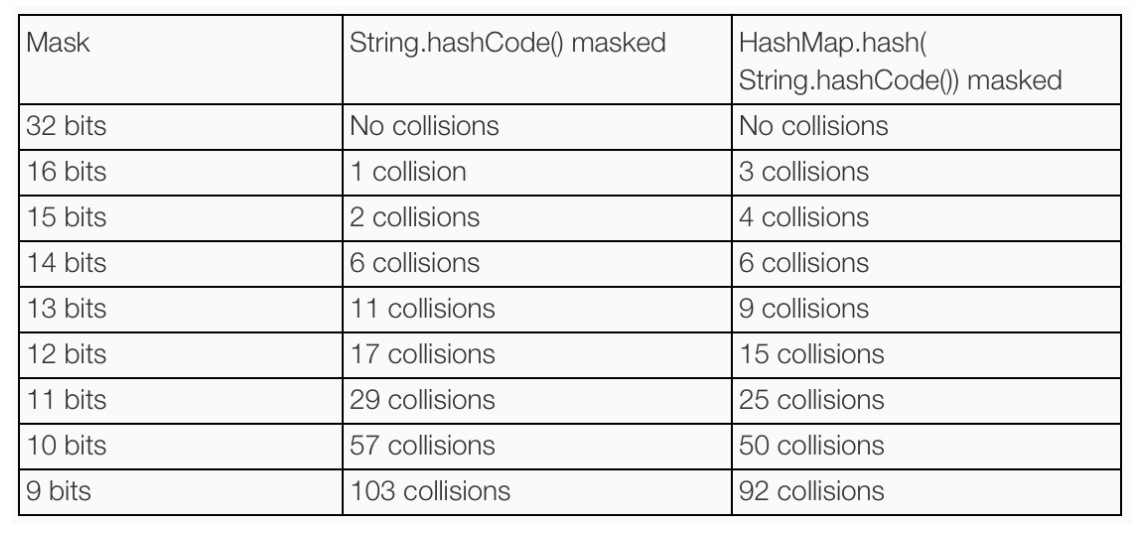https://www.todaysoftmag.com/article/1663/an-introduction-to-optimising-a-hashing-strategy

HashMap解决冲突使用拉链法。jdk1.8 中，当一个桶链表节点超过TREEIFY_THRESHOLD=8后，链表会转换为红黑树，当桶中节点移除或重新哈希少于 UNTREEIFY_THRESHOLD=6时，红黑树会转变为普通的链表。

HashMap 文档有这么一段描述。大体意思是，哈希桶上的链表节点数量呈现泊松分布

Ideally, under random hashCodes, the frequency of
* nodes in bins follows a Poisson distribution
* (http://en.wikipedia.org/wiki/Poisson_distribution) with a
* parameter of about 0.5 on average for the default resizing
* threshold of 0.75, although with a large variance because of
* resizing granularity. Ignoring variance, the expected
* occurrences of list size k are (exp(-0.5) * pow(0.5, k) /
* factorial(k)). The first values are:
*
* 0:    0.60653066
* 1:    0.30326533
* 2:    0.07581633
* 3:    0.01263606
* 4:    0.00157952
* 5:    0.00015795
* 6:    0.00001316
* 7:    0.00000094
* 8:    0.00000006
* more: less than 1 in ten million• $P$ 概率；
• $N$ 某种函数关系；
• $t$ 时间；
• $n$ 出现的数量；

/**
* @param n 节点数量
* @param r 平均数量
*/
public static String poisson(int n, double r) {
double value = Math.exp(-r) * Math.pow(r, n) / IntMath.factorial(n);
return new BigDecimal(value).setScale(10, ROUND_HALF_UP).toPlainString();
}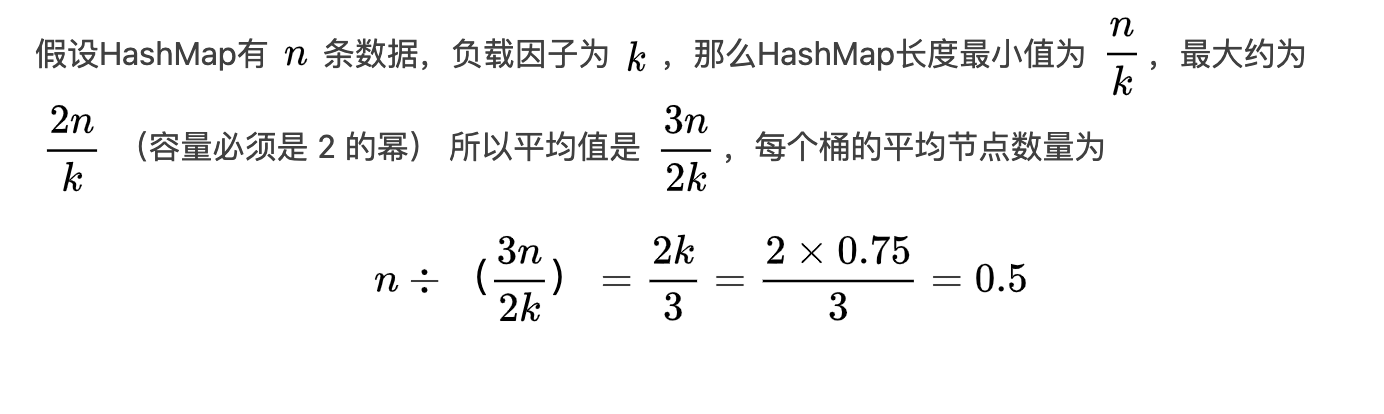HashMap 默认负载因子为 0.75，所以每个桶的平均节点数量 0.5，代入柏松公式得到下面数据

1个桶中出现1个节点的概率:0.3032653299
1个桶中出现2个节点的概率:0.0758163325
1个桶中出现3个节点的概率:0.0126360554
1个桶中出现4个节点的概率:0.0015795069
1个桶中出现5个节点的概率:0.0001579507
1个桶中出现6个节点的概率:0.0000131626
1个桶中出现7个节点的概率:0.0000009402
1个桶中出现8个节点的概率:0.0000000588